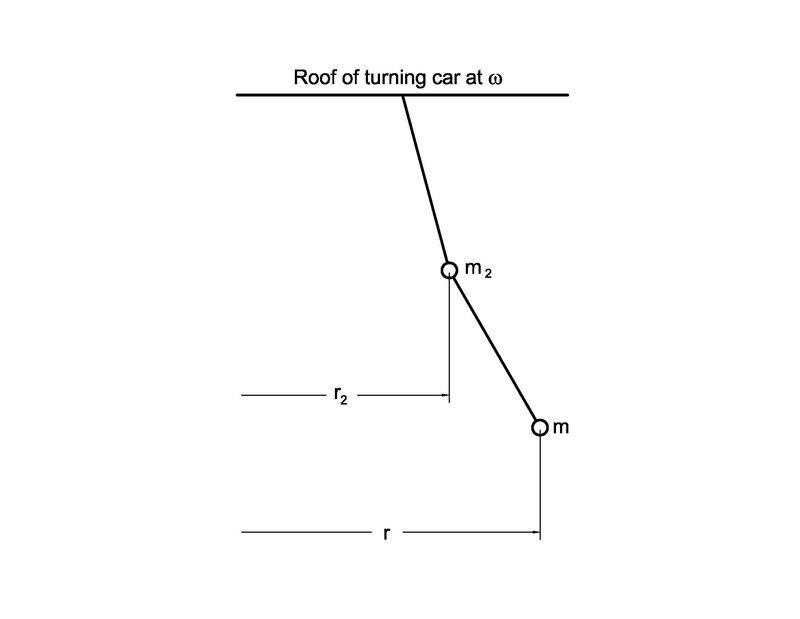# Hanging beads in vehicle turning on horizontal road (constant v and r)

• B
• AJG3

#### AJG3

TL;DR Summary
One hanging bead (massless string) in a turning car would deflect to angle atan(v^2/r*g) from vertical, but with a straight string, multiple beads are each at a different radius. So what is the curve of the string?
Neglect any forces within string, except tension.

Where are you stuck?

Welcome @AJG3 !
Also, could you show us a diagram showing the meaning of "but with a straight string, multiple beads are each at a different radius."

•nasu
Welcome @AJG3 !
Also, could you show us a diagram showing the meaning of "but with a straight string, multiple beads are each at a different radius."
Pretty sure he means:
• a bead at 2 inches along the string has a rotational radius of 2 inches
• a bead at 4 inches along the string has a rotational radius of 4 inches
• etc.

•Lnewqban
For specificity, I assumed n point mass beads, each with 1 unit of string length between. The last (outermost) will be bead 1, next inwards bead 2, and so forth I immediately realized that not only is each bead at a different radius, but also a different velocity, ω*ri, with ω the angular velocity of the car and all the beads. Let v and r apply to the outermost bead then ω = v/r.

The string supporting the outermost bead is at angle atan(v^2/rg) from vertical to balance forces including centripetal force. The tension in the string resolves into m*v^2/r horizontally and mg vertically.

r2 = r - sin(atan(v^2/rg) = r - (v^2/rg)/√(1+(v^2/rg)^2)
the vertical component of T supporting bead 2 must = 2*mg
the horizontal component of T supporting bead 2 must be mv2^2/r2+mv^2/r =
mω^2r2+mω^2r = mω^2(2r - (v^2/rg)/√(1+(v^2/rg)^2)))

so each successive centripetal force on the bead gets a bit stronger and the angles a bit farther from vertical, but beyond writing the second angle as an atan, I'm not seeing how to get the overall curve. It looks to me that the algebra explodes without major simplification.

Perhaps the answer is a continuous solution with no beads and distributed mass in the string. Since I always fail at deriving the catenary, I'm sure that problem would stump me too.

Pretty sure he means:
• a bead at 2 inches along the string has a rotational radius of 2 inches
• a bead at 4 inches along the string has a rotational radius of 4 inches
• etc.
Sorry. I can't draw a figure, but what I meant is that unless the beads are hanging vertically (they won't in a turning car), then each is at a different radius from the center of the car's circular path. Obviously, a sensible person makes the simplification that all the beads are within inches of each other and the car's turning radius is hundreds of feet, so the beads all have approximately the same turning radius and hang in a straight inclined line. But that is not the problem I'm trying to solve.

Sorry. I can't draw a figure, but what I meant is that unless the beads are hanging vertically (they won't in a turning car), then each is at a different radius from the center of the car's circular path.
Something like this?Thank you. That is it - looking forward for a left turn. And I realize I was wrong and the upper string would indeed be deflected less from vertical than the lower string: twice the gravitational force, but less than twice the centripetal force, since although it’s r is smaller, it’s v^2 is smaller still. mv^2/r = m* ω^2*r^2/r. So directly, not inversely proportional to r.

Reference: https://www.physicsforums.com/threa...l-road-constant-v-and-r.1047384/#post-6822920 ^2*r^2/r, so directly, not inversely, proportional to r.

•Lnewqban
Perhaps the answer is a continuous solution with no beads and distributed mass in the string. Since I always fail at deriving the catenary, I'm sure that problem would stump me too.
Yes, same for me.

My inclination would be to give up and go with a numerical approach for the finite case. Make an initial guess for a set of beads (say the straight line approximation) and compute the net force on each bead. Then relax the solution so that each bead moves in the direction of the net force, subject to the constraints imposed by the string. Iterate while a stable solution is approached.

This would be the "hill climbing" approach to optimization.

Graph the result and see if one can intuit an equation that fits the curve.